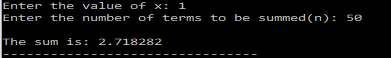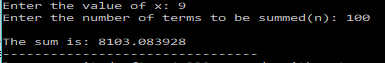# C Program to find the sum of a Finite Series – Tutorial

Finding the sum of a series(finite or infinite) is a crucial part of computational mathematics.

Many mathematical functions can be simply expressed in the form of a series as shown below:

Exponential Series:$e^x=1+x+\frac{x^2}{2!}+\frac{x^3}{3!}+...\frac{x^n}{n!}$

Sine Series:$\sin (x) = x - \frac{x^3}{3!} + \frac{x^5}{5!} - ....$

Cosine Series:$\cos (x) = 1 - \frac{x^2}{2!} + \frac{x^4}{4!} - ....$
NOTE: These can be obtained using the Taylor Series expansions.

In this post I will show you how to find the sum of a finite series efficiently.

Let’s consider the following series:$S(x)= 1 + x + \frac{x^2}{2!} + \frac{x^3}{3!} + ....$

Note: the no. of terms in this series is$(n+1)$

Now if you wanted to find the sum of the first 30 terms, then naively, you would try to run a loop and evaluate each term and thus find the sum. Though, doable, this method is not very efficient, especially if there are large numbers involved(like 20!).

A better way would be to find the ratio of consecutive terms and then use that to calculate the successive terms of the series and thus find the sum. This would eliminate the problem of needlessly working with large numbers, as the ratio of successive terms   would be relatively very small.

The approach would be something like this:

Find the ratio of the successive terms,$R=\frac{t_i}{t_{i-1}}=\frac{x}{i}$

Now, using this, the successive terms can be found as:$t_1=R.t_0$$t_2=R.t_1$
….. and so on.

For a program to find the sum for the first n terms can be written as shown below:

### PROGRAM:

/********************************
******FINITE SERIES SUM**********
Series: S(x) = 1 + x + x/1 + (x^2/2!) + (x^3/3!) + ..... + (x^n/n!)
********************************/
#include<stdio.h>
#include<math.h>
main(){
int i,n;
/*Initialize t0 with the value of the first term of the series */
double t0=1;
/*Declare more variables to be used */
double x;
double t1,R,sum=t0;
printf("Enter the value of x: ");
scanf("%lf",&x);
printf("Enter the number of terms to be summed(n): ");
scanf("%d",&n);
for(i=1;i<=n;i++){
R=(double)x/i;
t1=R*t0;
t0=t1;
sum=sum+t1;
}
printf("\nThe sum is: %f",sum);
}


As you might have noticed by now, the above series is the exponential series, and you can check your results calculating the exponential of the function.

### OUTPUT: